Linear Regression
A linear regression is also know as the "line of best fit".

Side note:  Although commonly used when dealing with "sets" of data, the linear regression can also be used to simply find the equation of the line between two points.
Example: Find the equation of the line passing through (-1, 1) and (-4,7).
Entering the information as shown in the screens below, we arrive at the equation of the line: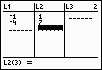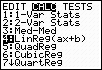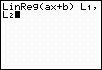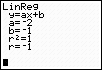The equation is y = -2x -1. The correlation coefficient is -1 since both point are "on" the line and the line slopes negatively.

Linear Regression Model Example

Let's examine an example of the linear regression as it pertains to a "set" of data.

Data:  Is there a relationship between Math SAT scores and the number of hours spent studying for the test?  A study was conducted involving 20 students as they prepared for and took the Math section of the SAT Examination.Task: a.) Determine a linear regression model equation to represent this data. b.) Graph the new equation. c.) Decide whether the new equation is a "good fit" to represent this data. d.) Interpolate data:  If a student studied for 15 hours, based upon this study, what would be the expected Math SAT score?
Hours Spent Studying
Math SAT Score
4
390
9
580
10
650
14
730
4
410
7
530
12
600
22
790
1
350
3
400
8
590
11
640
5
450
6
520
10
690
11
690
16
770
13
700
13
730
10
640
 e.) Interpolate data:  If a student obtained a Math SAT score of 720, based upon this study, how many hours did the student most likely spend studying? f.) Extrapolate data:  If a student spent 100 hours studying, what would be the expected Math SAT score?  Discuss this answer. Any answers in relation to this problem are to be rounded to the nearest tenth. If rounding is not indicated in a problem, leave the full calculator entries as answers.
Step 1.  Enter the data into the lists.
For basic entry of data, see Basic Commands.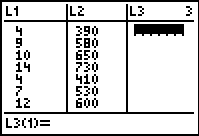Step 2.  Create a scatter plot of the data.
Go to STATPLOT (2nd Y=) and choose the first plot.  Turn the plot ON, set the icon to Scatter Plot (the first one), set Xlist to L1 and Ylist to L2 (assuming that is where you stored the data), and select a Mark of your choice.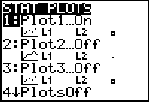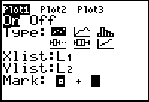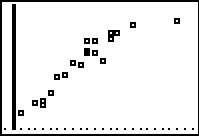Step 3.  Choose Linear Regression Model.
Press STAT, arrow right to CALC, and arrow down to 4: LinReg (ax+b).  Hit ENTER.  When LinReg appears on the home screen, type the parameters L1, L2, Y1.  The Y1 will put the equation into Y= for you.
(Y1 comes from VARS → YVARS, #Function, Y1)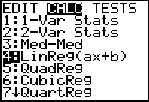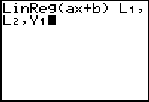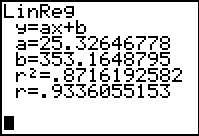The linear regression equation is
y = 25.3x + 353.2

Step 4.  Graph the Linear Regression Equation from Y1.

ZOOM #9 ZoomStat to see the graph.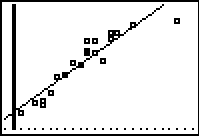Step 5.  Is this model a "good fit"?
The correlation coefficient, r, is .9336055153 which places the correlation into the "strong" category.  (0.8 or greater is a "strong" correlation)
The coefficient of determination, r 2, is .8716192582 which means that 87% of the total variation in y can be explained by the relationship between x and y.  The other 13% remains unexplained.
Yes, it is a "good fit".Step 6.  Interpolate:  (within the data set)

If a student studied for 15 hours, based upon this study, what would be the expected Math SAT score?

From the graph screen, hit TRACE, arrow up to obtain the linear equation at the top of the screen, type 15, hit ENTER, and the answer will appear at the bottom of the screen.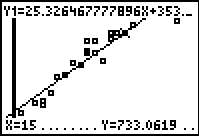Math SAT score of 733.1)
Step 7.  Interpolate: (within the data set)
If a student obtained a Math SAT score of 720, based upon this study, how many hours did the student most likely spend studying?

Go to TBLSET (above WINDOW) and set the TblStart to 13 (since 13 hours gives a score of 700).  Set the delta Tbl to a decimal setting of your choice.  Go to TABLE (above GRAPH) and arrow up or down to find your desired score of 720, in the Y1 column.
;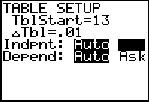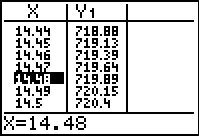(
answer to part e --  approx. 14.5 hours)
Step 8.  Extrapolate data:  (beyond the data set)
If a student spent 100 hours studying, what would be the expected Math SAT score?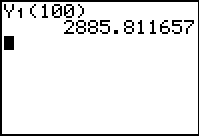With your linear equation in Y1, go to the home screen and type Y1(100).  Press ENTER. Our equation shows that if a student studies 100 hours, he/she should score 2885.8 on the Math section of the SAT examination.  The only problem with this answer is that the highest score that can be obtained is 800.  So why is this score so outrageous?   ANSWER:  When you extrapolate data, the further you move away from the data set, the less accurate your information becomes.  In this problem, the largest number of hours in the data set was 22 hours, but the extrapolation tried to jump to 100 hours. (answer to part f)